# Matlab的搜索路径

Matlab共有两种搜索路径

• MATLAB提供的文件夹或者其它MathWorks产品
这些文件夹在 “matlabroot/toolbox“下, 使用matlabroot 可以查看该根目录.

• MATLAB用户路径（userpath）
用户路径文件夹是存储那些MATLAB在启动时添加到搜索路径的文件.

# MATLAB搜索路径的添加

## 使用图形用户界面添加搜索路径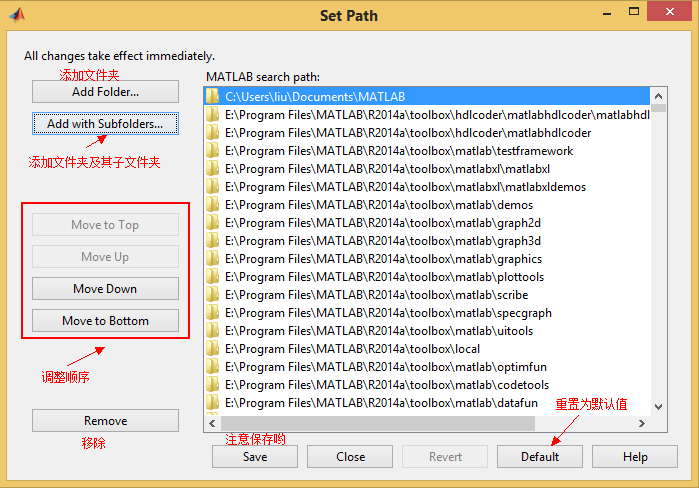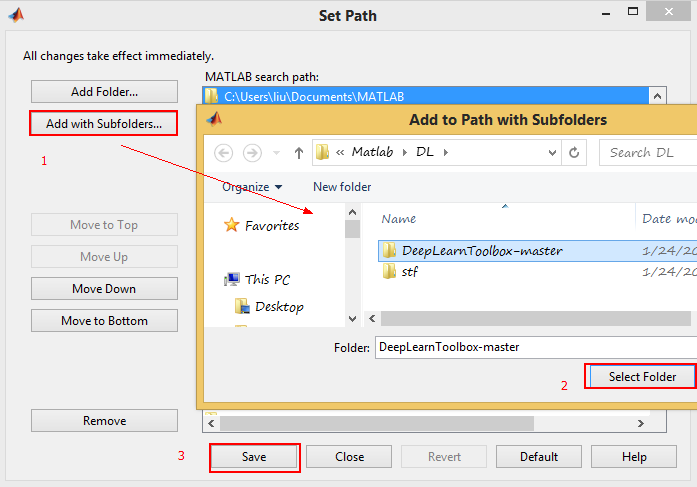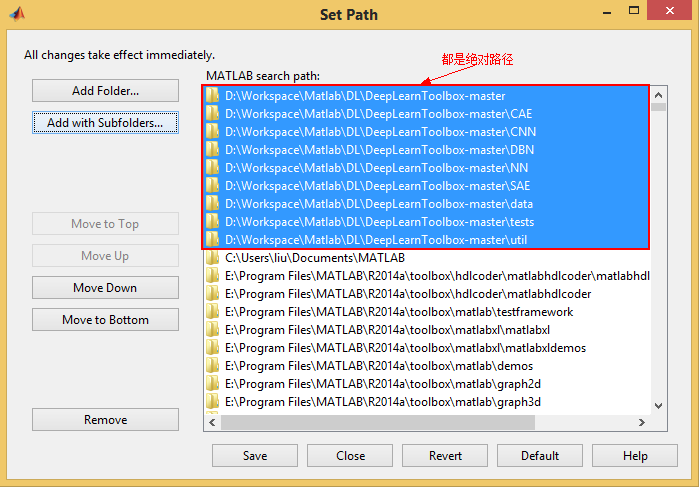## 使用命令添加搜索路径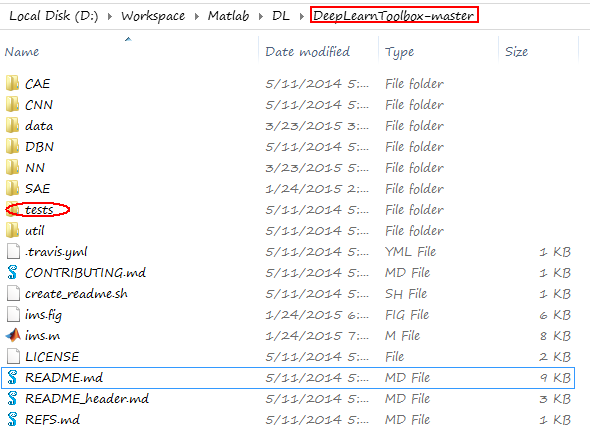addpath('D:\Workspace\Matlab\DL\DeepLearnToolbox-master');

addpath('D:\Workspace\Matlab\DL\DeepLearnToolbox-master','-end');

### 添加绝对路径

genpath('D:\Workspace\Matlab\DL\DeepLearnToolbox-master')

ans =

D:\Workspace\Matlab\DL\DeepLearnToolbox-master;D:\Workspace\Matlab\DL\DeepLearnToolbox-master\CAE;D:\Workspace\Matlab\DL\DeepLearnToolbox-master\CNN;D:\Workspace\Matlab\DL\DeepLearnToolbox-master\DBN;D:\Workspace\Matlab\DL\DeepLearnToolbox-master\NN;D:\Workspace\Matlab\DL\DeepLearnToolbox-master\SAE;D:\Workspace\Matlab\DL\DeepLearnToolbox-master\data;D:\Workspace\Matlab\DL\DeepLearnToolbox-master\tests;D:\Workspace\Matlab\DL\DeepLearnToolbox-master\util;

addpath(genpath('D:\Workspace\Matlab\DL\DeepLearnToolbox-master'));

### 添加相对路径

addpath(genpath('DeepLearnToolbox'));

ans =

DeepLearnToolbox-master;DeepLearnToolbox-master\CAE;DeepLearnToolbox-master\CNN;DeepLearnToolbox-master\DBN;DeepLearnToolbox-master\NN;DeepLearnToolbox-master\SAE;DeepLearnToolbox-master\data;DeepLearnToolbox-master\tests;DeepLearnToolbox-master\util;

# MATLAB搜索路径的移除

### 移除单个路径

• 对于绝对路径, 那么只需输入下面的命令
rmpath('D:\Workspace\Matlab\DL\DeepLearnToolbox-master')
• 对于相对路径, 那么需输入下面的命令, 否则, 提示找不到.
rmpath('DeepLearnToolbox-master')

### 移除多个路径

• 对于绝对路径, 那么只需输入下面的命令
rmpath(genpath('D:\Workspace\Matlab\DL\DeepLearnToolbox-master'))
• 对于相对路径, 那么需输入下面的命令
rmpath(genpath('DeepLearnToolbox-master'))

### 还有一种方法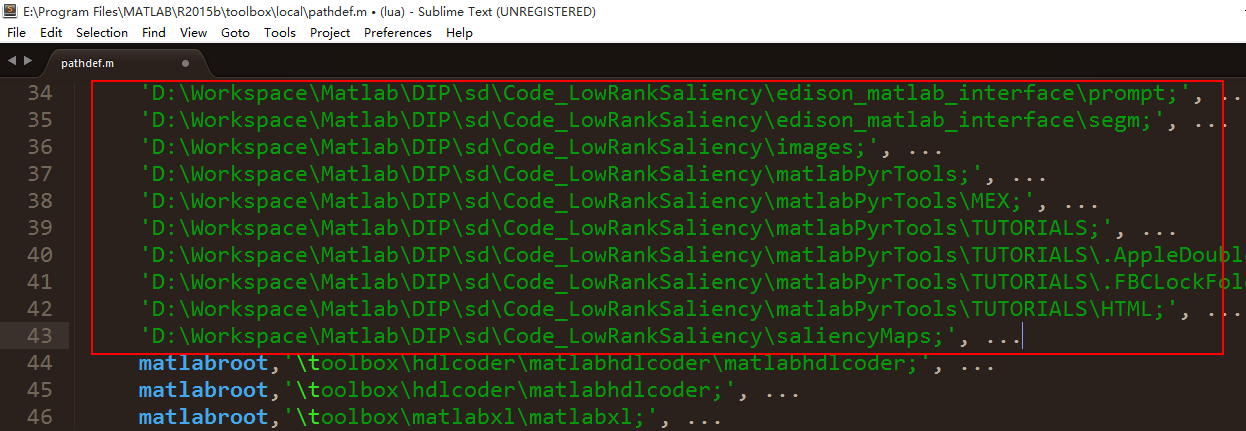©️2019 CSDN 皮肤主题: 大白 设计师: CSDN官方博客